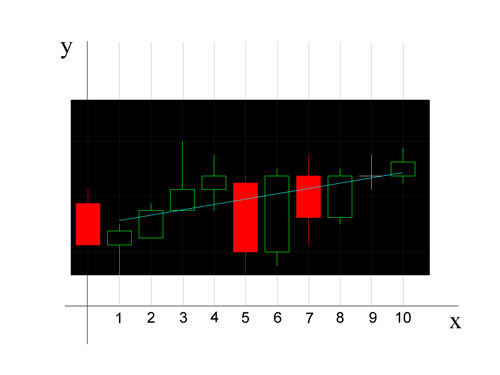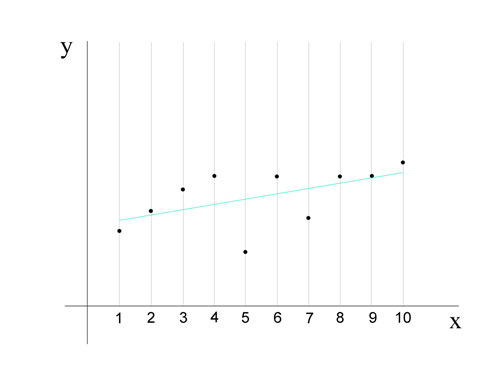# Tutorial 48 | Creating a linear regression program

Welcome to tutorial 48 in this series of tutorials designed to teach EasyLanguage programming skills. This tutorial applies to TradeStation 8.7 and newer versions, as well as to MultiCharts.

In this tutorial we create and test a show me study that creates a line using linear regression that best fits chart data. The linear regression method used in this tutorial uses the least squares method to calculate the slope and y-intercept of this line.

The formulae we use are as follows:

Slope(m) = ( NΣXY – ( ΣX )( ΣY ) ) / ( NΣX2 – ( ΣX ))

Intercept(c) = ( ΣY – m( ΣX ) ) / N

Equation(y) = mx + c

Where the ‘Σ‘ symbol means ‘sum.’

In the tutorial, we first get closing data for the most recent ten bars. We then use the above formulae to calculate the slope and y intercept using a spreadsheet. We finally demonstrate the calculation in TradeStation and draw a line.The above graph shows a part of a TradeStation chart applied over an x – y graph.This graph is the same as the previous one except it just shows the closing values

Please join our email mailing list if you have not already done so and we will let you know when we release new tutorials or programs.

## Tutorial 48 Video 1 – Introduction to linear regression formulae and spreadsheet calculation

Correction: Make sure that you add the following code: SumY = 0; SumXY = 0; immediately after the print statement.